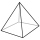# Cone A2V

Surface of cone in the plane is a circular arc with central angle of 126° and area 415 cm2.

Calculate the volume of a cone.

Result

V =  881.1 cm3

#### Solution:Leave us a comment of this math problem and its solution (i.e. if it is still somewhat unclear...):Be the first to comment!#### Following knowledge from mathematics are needed to solve this word math problem:

Need help calculate sum, simplify or multiply fractions? Try our fraction calculator. Pythagorean theorem is the base for the right triangle calculator.

## Next similar math problems:

1. Axial sectionAxial section of the cone is an equilateral triangle with area 208 dm2. Calculate the volume of the cone.
2. Tetrahedral pyramidWhat is the surface of a regular tetrahedral (four-sided) pyramid if the base edge a=16 and height v=16?
3. Trapezoid MOThe rectangular trapezoid ABCD with right angle at point B, |AC| = 12, |CD| = 8, diagonals are perpendicular to each other. Calculate the perimeter and area of ​​the trapezoid.
4. The tractorThe tractor sows an average of 1.5 ha per hour. In how many hours does it sows a rectangular trapezoid field with the bases of 635m and 554m and a longer arm 207m?
5. Company logoThe company logo consists of a blue circle with a radius of 4 cm, which is an inscribed white square. What is the area of the blue part of the logo?
6. Area of a rectangleCalculate the area of a rectangle with a diagonal of u = 12.5cm and a width of b = 3.5cm. Use the Pythagorean theorem.
7. GardenArea of a square garden is 6/4 of triangle garden with sides 56 m, 35 m, and 35 m. How many meters of fencing need to fence a square garden?
8. Cube in a sphereThe cube is inscribed in a sphere with volume 9067 cm3. Determine the length of the edges of a cube.
9. CuboidCuboid with edge a=16 cm and body diagonal u=45 cm has volume V=11840 cm3. Calculate the length of the other edges.
10. Triangle SASCalculate the area and perimeter of the triangle, if the two sides are 51 cm and 110 cm long and angle them clamped is 130 °.
11. RectangleThe rectangle is 21 cm long and 38 cm wide. Determine the radius of the circle circumscribing rectangle.
12. Trigonometric functionsIn right triangle is: ? Determine the value of s and c: ? ?
13. Right ΔA right triangle has the length of one leg 7 cm and length of the hypotenuse 25 cm. Calculate the height of the triangle.
14. Circle chordWhat is the length d of the chord circle of diameter 50 dm, if the distance from the center circle is 21 dm?
15. Distance between 2 pointsFind the distance between the points (7, -9), (-1, -9)
16. Right pyramidA right pyramid on a base 4 cm square has a slant edge of 6 cm. Calculate the volume of the pyramid.
17. MovementFrom the crossing of two perpendicular roads started two cyclists (each at different road). One runs at average speed 28 km/h, the second at average speed 24 km/h. Determine the distance between them after 45 minutes cycling.